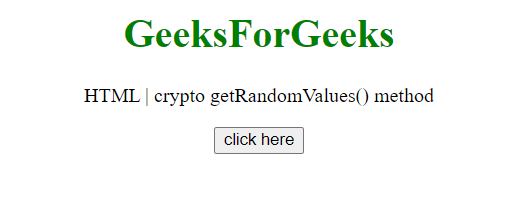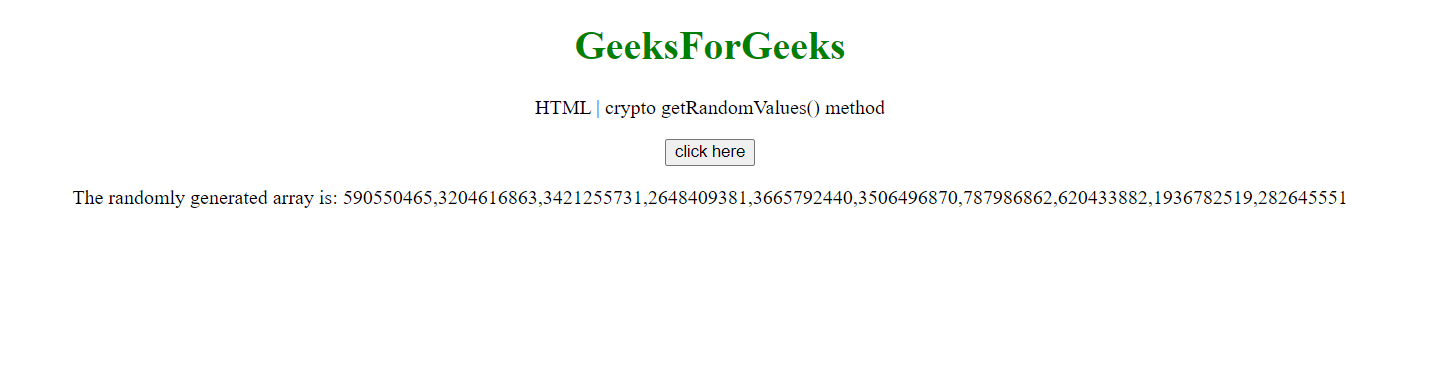Related Articles

# HTML DOM crypto.getRandomValues() method

• Last Updated : 16 Jul, 2020

The crypto getRandomValues() method lets you get cryptographic random values. The array given as the parameter to this method gets filled with cryptographic random numbers.

Syntax:

Hey geek! The constant emerging technologies in the world of web development always keeps the excitement for this subject through the roof. But before you tackle the big projects, we suggest you start by learning the basics. Kickstart your web development journey by learning JS concepts with our JavaScript Course. Now at it's lowest price ever!

`var a = window.crypto.getRandomValues(Array);`

Parameters:

• Array: An integer-based Array, can be Int8Array, UInt8Array, Int16Array, Uint16Array, Int32Array, or Uint32Array. All elements in the array are overwritten with random numbers after using this method.

Return Value: The Array passed in this method is overwritten and returned.

Example: This example uses the getRandomValues() method to generate a random Uint32Array of size 10.

 ` ``<``html``>  ``<``head``>``    ``<``title``>crypto getRandomValues() method``   ``<``body` `style``=``"text-align:center;"``>``    ``<``h1` `style``=``"color:green;"``>  ``        ``GeeksForGeeks  ``    `` ``    ``<``p``> ``    ``HTML | crypto getRandomValues() method    ``    ````    ``<``button` `onclick` `= ``"Geeks();"``>``    ``click here``    ````    ``<``p` `id``=``"arr"``> ``    ``       ``    ``<``script``> ``        ``var arr = document.getElementById("arr");``        ``function Geeks() {``            ``var array = new Uint32Array(10);``            ``a = window.crypto.getRandomValues(array);``            ``console.log(a);``            ``arr.innerHTML = ``           ``"The randomly generated array is: "+a;``        ``} ``    `` ``   ```

Output:

Before Clicking Button:After Clicking Button:Supported Browsers: